Post

# C# Heap(ing) Vs Stack(ing) in .NET: Part II

• 251.1k
• 0
• 5

Even though with the .NET framework we don't have to actively worry about memory management and garbage collection (GC), we still have to keep memory management and GC in mind in order to optimize the performance of our applications. Also, having a basic understanding of how memory management works will help explain the behavior of the variables we work with in every program we write. In this article I'll cover some of the behaviors we need to be aware of when passing parameters to methods.

In Part I we covered the basics of the Heap and Stack functionality and where Variable Types and Reference Types are allocated as our program executes. We also covered the basic idea of what a Pointer is.

Parameters, the Big Picture.

Here's the detailed view of what happens as our code executes. We covered the basics of what happens when we make a method call in Part I. Let's get into more detail...

When we make a method call here's what happens:

1. Space is allocated for information needed for the execution of our method on the stack (called a Stack Frame). This includes the calling address (a pointer) which is basically a GOTO instruction so when the thread finishes running our method it knows where to go back to in order to continue execution.
2. Our method parameters are copied over. This is what we want to look at more closely.
3. Control is passed to the JIT'ted method and the thread starts executing code. Hence, we have another method represented by a stack frame on the "call stack".

The code:

{
int result;
result = pValue + 5;
return result;

}

Will make the stack look like this: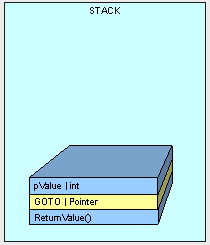NOTE : the method does not live on the stack, and is illustrated here just for reference as the beginnnig of the stack frame.

As discussed in Part I, Parameter placement on the stack will be handled differently depending on whether it is a value type or a reference type. A value types is copied over and the reference of a reference type is copied over.ed over.

Passing Value Types.

Here's the catch with value types...

First, when we are passing a value types, space is allocated and the value in our type is copied to the new space on the stack. Look at the following method:

class Class1

{

public void Go()

{

int x = 5;

Console.WriteLine(x.ToString());

}

{

pValue += 5;

return pValue;

}

}

As the method executes, space for "x" is placed on the stack with a value of 5.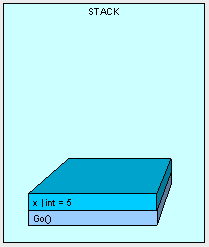Next, AddFive() is placed on the stack with space for it's parameters and the value is copied, bit by bit from x.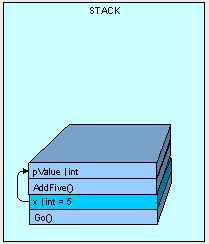When AddFive() has finished execution, the thread is passed back to Go() and because AddFive() has completed, pValue is essentially "removed":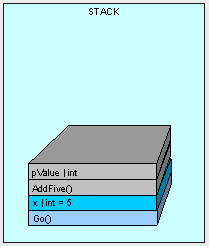So it makes sense that the output from our code is "5", right? The point is that any value type parameters passed into a method are carbon copies and we count on the original variable's value to be preserved.

One thing to keep in mind is that if we have a very large value type (such as a big struct) and pass it to the stack, it can get very expensive in terms of space and processor cycles to copy it over each time. The stack does not have infinite space and just like filling a glass of water from the tap, it can overflow. A struct is a value type that can get pretty big and we have to be aware of how we are handling it.

Here's a pretty big struct:

public struct MyStruct

{

long a, b, c, d, e, f, g, h, i, j, k, l, m;

}

Take a look at what happens when we execute Go() and get to the DoSomething() method below:

public void Go()

{

MyStruct x = new MyStruct();

DoSomething(x);

}

public void DoSomething(MyStruct pValue)

{

// DO SOMETHING HERE....

}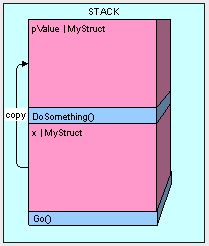This can be really inefficient. Imaging if we passed the MyStruct a couple thousand times and you can understand how it could really bog things down.

So how do we get around this problem? By passing a reference to the original value type as follows:

public void Go()

{

MyStruct x = new MyStruct();

DoSomething(ref x);

}

public struct MyStruct

{

long a, b, c, d, e, f, g, h, i, j, k, l, m;

}

public void DoSomething(ref MyStruct pValue)

{

// DO SOMETHING HERE....

}

This way we end up with more memory efficient allocation of our objects in memory.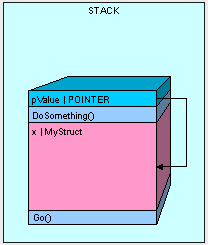The only thing we have to watch out for when passing our value type by reference is that we have access to the value type's value. Whatever is changed in pValue is changed in x. Using the code below, our results are going to be "12345" because the pValue.a actually is looking at the memory space where our original x variable was declared.

public void Go()

{

MyStruct x = new MyStruct();

x.a = 5;

DoSomething(ref x);

Console.WriteLine(x.a.ToString());

}

public void DoSomething(ref MyStruct pValue)

{

pValue.a = 12345;

}

Passing Reference Types.

Passing parameters that are reference types is similar to passing value types by reference as in the previous example.

If we are using the value type

public class MyInt

{

public int MyValue;

}

And call the Go() method, the MyInt ends up on the heap because it is a reference type:

public void Go()

{

MyInt x = new MyInt();

}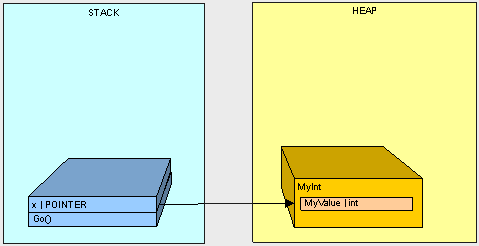If we execute Go() as in the following code ...

public void Go()

{

MyInt x = new MyInt();

x.MyValue = 2;

DoSomething(x);

Console.WriteLine(x.MyValue.ToString());

}

public void DoSomething(MyInt pValue)

{

pValue.MyValue = 12345;

}

Here's what happens...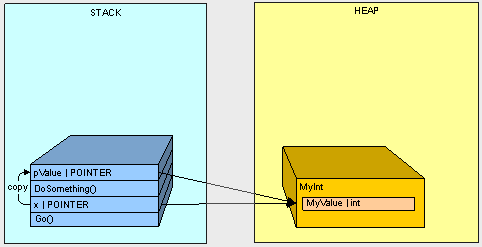1.  Starting with the call to Go() the variable x goes on the stack.
2. Starting with the call to DoSomething() the parameter pValue goes on the stack.
3. The value of x (the address of MyInt on the stack) is copied to pValue

So it makes sense that when we change the MyValue property of the MyInt object in the heap using pValue and we later refer to the object on the heap using x, we get the value "12345".

So here's where it gets interesting. What happens when we pass a reference type by reference?

Check it out. If we have a Thing class and Animal and Vegetables are both things:

public class Thing

{

}

public class Animal:Thing

{

public int Weight;

}

public class Vegetable:Thing

{

public int Length;

}

And we execute the Go() method below:

public void Go()

{

Thing x = new Animal();

Switcharoo(ref x);

Console.WriteLine(

"x is Animal    :   "

+ (x is Animal).ToString());

Console.WriteLine(

"x is Vegetable :   "

+ (x is Vegetable).ToString());

}

public void Switcharoo(ref Thing pValue)

{

pValue = new Vegetable();

}

Our variable x is turned into a Vegetable.

x is Animal    :   False
x is Vegetable :   True

Let's take a look at what's happening:1. Starting with the Go() method call, the x pointer goes on the stack
2. The Animal goes on the hea
3. Starting with the call to Switcharoo() method, the pValue goes on the stack and points to x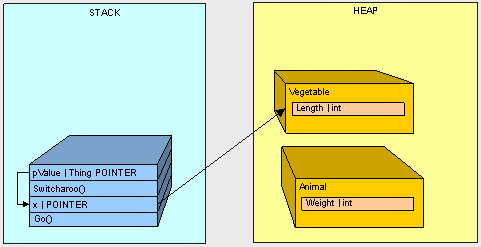4. The Vegetable goes on the heapthe heap
5. The value of x is changed through pValue to the address of the Vegetable

If we don't pass the Thing by ref, we'll keep the Animal and get the opposite results from our code.

If the above code doesn't make sense, check out my article on types of Reference variables to get a better understanding of how variables work with reference types.

In Conclusion.

We've looked at how parameter passing is handled in memory and now know what to look out for. In the next part of this series, we'll take a look at what happens to reference variables that live in the stack and how to overcome some of the issues we'll have when copying objects.

For now.

-Happy Coding

Part I | Part II | Part III | Part IV

Recommended Free Ebook
Similar Articles